Author: Oscar Cronquist Article last updated on March 05, 2021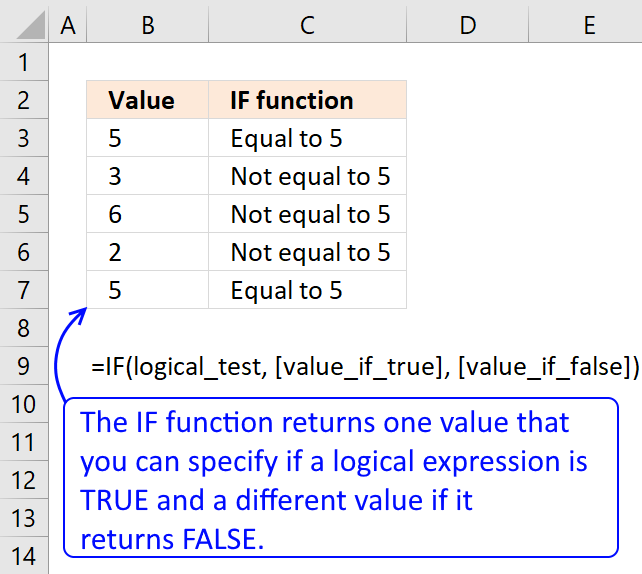The IF function returns one value if the logical test is TRUE and another value if the logical test is FALSE.

Excel function syntax

IF(logical_test, [value_if_true], [value_if_false])

Arguments

 logical_test Required. The logical expression determines what value the IF function returns.  Excel evaluates all numerical values positive or negative to TRUE except 0 (zero) that equals FALSE. [value_if_true] Optional. The value the IF function returns if the logical expression evaluates to TRUE. If omitted 0 (zero) is returned. [value_if_false] Optional. The value the IF function returns if the logical expression evaluates to FALSE. If omitted 0 (zero) is returned.

Comment

The IF function is one of the most used functions in Excel.

1. What is a logical expression?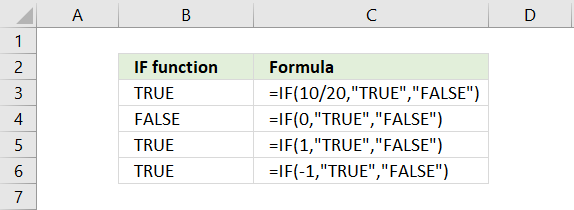The logical_test argument in the IF function determines the outcome meaning if the second argument [value_if_true] or third argument [value_if_false] will be returned or calculated.

Boolean value TRUE or FALSE are valid outcomes, however, their numerical equivalents are also valid. For example, 0 (zero) is evaluated as FALSE and all other numerical values, even negative values, are evaluated as TRUE.

1.1 Can a decimal number be used as a logical expression?Formula in cell B3:

=IF(10/20, "TRUE", FALSE")

The logical_test argument in the formula above is 10/20 which equals 0.5. 0.5 is not equal to 0 (zero) so the formula returns TRUE in cell B3.

1.2 Is 0 (zero) equivalent to boolean value FALSE in a logical expression?Formula in cell B4:

=IF(0, "TRUE", FALSE")

The logical_test argument in cell B4 above is 0 (zero). 0 (zero) equals 0 (zero) so the formula returns FALSE in cell B3.

1.3 Is 1 equivalent to boolean value TRUE in a logical expression?Formula in cell B5:

=IF(1, "TRUE", FALSE")

The logical_test argument in the formula above is 1. 1 is not equal to 0 (zero) so the formula returns TRUE in cell B3.

1.4 Is -1 (negative number) equivalent to boolean value TRUE in a logical expression?Formula in cell B6:

=IF(-1, "TRUE", FALSE")

The logical_test argument in cell B6 above is -1. -1 is not equal to 0 (zero) and the formula returns TRUE in cell B3.

1.5 What happens if a text string is used in the logical expression?=IF("A", TRUE, FALSE)

The formula above returns #VALUE! error which means that text strings are not allowed as a logical test, however a logical expression using text strings are fine, see example below.

=IF("A"="A", TRUE, FALSE)

This formula will return TRUE because "A" is equal to "A".

2. What logical operators can you use in the logical expression?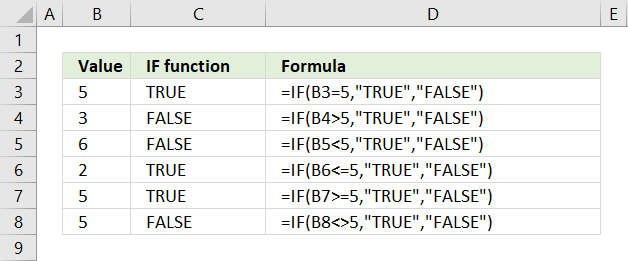The logical_test argument allows you to use comparison operators, these characters let you to do more advanced comparisons than the equal sign.

• = (equal sign)
• < less than
• > greater than
• <= less than or equal to
• >= greater than or equal to
• <> not equal

Formula in cell C3:

=IF(B3=5, TRUE, FALSE)

This formula compares the value in cell B3 with number 5, if equal TRUE is returned. Note that this formula is only for demonstration purposes, you can use this formula in cell C3 to get the same result =B3=5.

If you change the value in cell B3 the formula in cell C3 instantly recalculates and returns TRUE or FALSE based on the outcome of the logical_test.

Formula in cell C4:

=IF(B4>5, TRUE, FALSE)

The formula in cell C4 checks if the value in cell B4 is larger than 5, cell B4 contains 3 and 3 is not larger than 5 so the formula returns FALSE.

Formula in cell C5:

=IF(B5<5, TRUE, FALSE)

This formula evaluates if the value in cell B5 is smaller than 5, the image above shows that cell B5 contains number 6. 6 is not smaller than 5 and the formula returns FALSE.

Formula in cell C6:

=IF(B6<=5, TRUE, FALSE)

The formula in cell C6 evaluates if the value in cell B6 is smaller than or equal to 5. Cell B6 contains 2, see image above, and 2 is smaller than or equal to 5. The formula returns TRUE in cell C6.

Formula in cell C7:

=IF(B7>=5, TRUE, FALSE)

This formula checks if value in cell B7 is larger than or equal to 5, cell B7 contains 5 and 5 is equal to 5. The formula returns TRUE in cell C7.

Formula in cell C8:

=IF(B8<>5, TRUE, FALSE)

The formula in cell C8 assesses if value in cell B8 is not equal to 5, cell B8 contains 5. 5 is equal to 5 and the formula returns FALSE in cell C8.

3. Use an Excel function to perform a logical test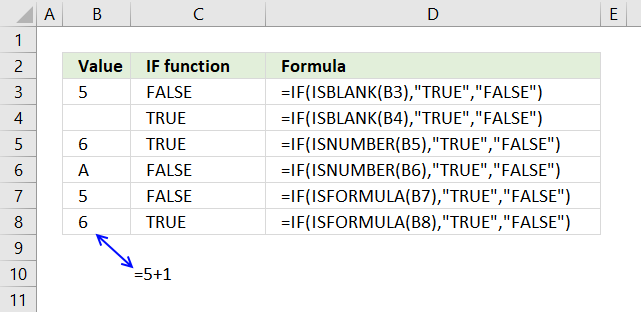You are not limited to comparison operators when dealing with the logical_test argument, any function that returns TRUE or FALSE can be used.

=IF(ISBLANK(B3),TRUE, FALSE)

The picture above shows a formula in cell C3 that evaluates if cell B3 is blank. It is not blank so it returns FALSE.

=IF(ISBLANK(B4),TRUE, FALSE)

Cell B4 is blank and the IF function returns TRUE in cell C4.

The following formula uses the ISNUMBER function to identify the value in cell B5.

=IF(ISNUMBER(B5),TRUE, FALSE)

The formula in cell C5 returns TRUE because the value in cell B5 is 6 and that is a number.

=IF(ISNUMBER(B6),TRUE, FALSE)

Cell C7 and C8 contain formulas that test if B7 and B8 contain a formula. Formula in cell C8 returns TRUE, cell B8 contains this formula =5+1.

Check out the functions in the Information category, many of them return TRUE or FALSE.

4. IF function - How to check if a cell is blank?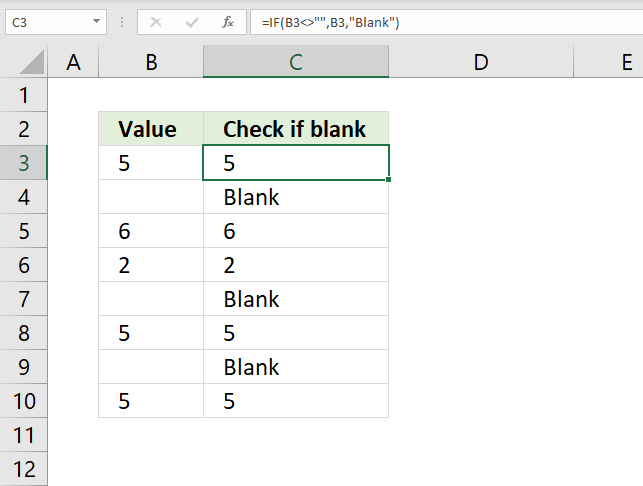The image above demonstrates the IF function in cell C3. It checks if the adjacent cell on the same row in column B is blank. If not blank the value itself is returned.

Formula in cell C3:

=IF(B3="","Blank",B3)

Cell reference B3 is a relative cell reference meaning it changes accordingly when you copy cell C3 and paste it to cells below.

Explaining formula in cell C3

Step 1 - Logical expression

The logical expression contains the equal sign. It checks if one value is equal to another value and returns boolean values TRUE or FALSE.

"" is the same as nothing or an empty cell.

B3=""

becomes

5=""

and returns FALSE. 5 is not equal to nothing.

Step 2 - Evaluate IF function

The second argument in the IF function is returned if the logical expression returns TRUE and the third is returned if FALSE.

IF(logical_test, [value_if_true], [value_if_false])

IF(B3="","Blank",B3)

becomes

IF(FALSE,"Blank",B3)

becomes

IF(FALSE,"Blank",5)

and returns 5 or the value itself in cell B3.

5. IF function - How to check if a cell is not blank?The image above demonstrates the IF function in cell C3. It checks if the adjacent cell on the same row in column B is not blank. If not blank the value itself is returned.

Formula in cell C3:

=IF(B3<>"",B3,"Blank")

Cell reference B3 is a relative cell reference meaning it changes accordingly when you copy cell C3 and paste it to cells below.

Explaining formula in cell C3

Step 1 - Logical expression

The logical expression contains two logical operaters, the first one is smaller than and the seconmd is larger than. When you combine these you get not equal to.

"" is the same as nothing or an empty cell.

B3<>""

becomes

5<>""

and returns TRUE. 5 is not equal to nothing.

Step 2 - Evaluate IF function

The second argument in the IF function is returned if the logical expression returns TRUE and the third is returned if FALSE.

IF(logical_test, [value_if_true], [value_if_false])

IF(B3<>"",B3,"Blank")

becomes

IF(TRUE,B3,"Blank")

becomes

IF(TRUE,5,"Blank")

and returns 5 or the value itself in cell B3.

6. IF function - How to check if a cell contains an error?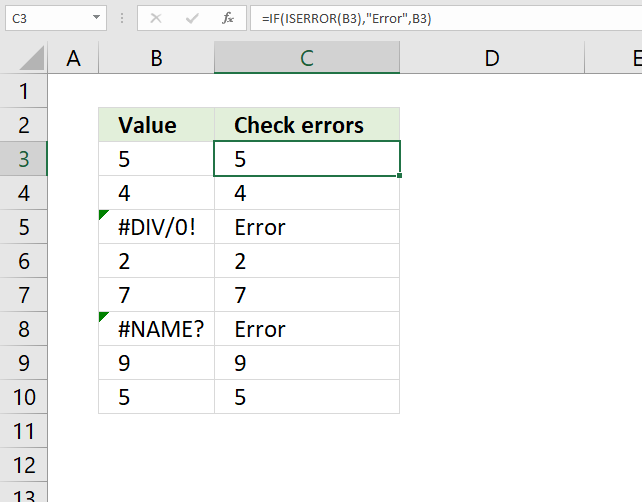The image above demonstrates an IF function in cell C3. It checks if the adjacent cell on the same row in column B contains an error value. The formula returns "Error" if true and the value itself if false.

Formula in cell C3:

=IF(ISERROR(B3), "Error", B3)

Cell reference B3 is a relative cell reference meaning it changes accordingly when you copy cell C3 and pastes it to cells below.

Explaining formula in cell C3

Step 1 - Logical expression

The ISERROR function returns TRUE if the cell contains an error and FALSE if not.

ISERROR(B3)

becomes

ISERROR(5)

and returns FALSE.

Step 2 - Evaluate IF function

The second argument in the IF function is returned if the logical expression returns TRUE and the third is returned if FALSE.

IF(logical_test, [value_if_true], [value_if_false])

IF(ISERROR(B3), "Error", B3)

becomes

IF(FALSE, "Error", B3)

becomes

IF(FALSE, "Error", 5)

and returns 5.

7. IF function - How to check if a cell is a text value?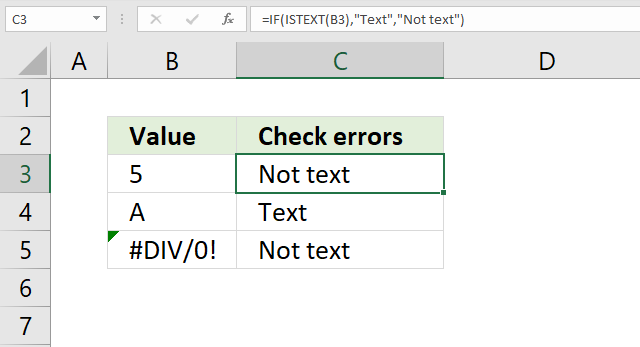The image above shows an IF function in cell C3 that checks if the adjacent cell on the same row in column B is a text value. The formula returns "Text" if true and "Not text" if false.

Formula in cell C3:

=IF(ISTEXT(B3),"Text","Not text")

Cell reference B3 is a relative cell reference meaning it changes accordingly when you copy cell C3 and pastes it to cells below.

Explaining formula in cell C3

Step 1 - Logical expression

ISTEXT(B3)

becomes

ISTEXT(5)

and returns boolean FALSE.

Step 2 - Evaluate IF function

The ISTEXT function returns True if the argument is text and False if not.

IF(ISTEXT(B3), "Text", "Not text")

becomes

IF(False, "Text", "Not text")

and returns "Not text" in cell C3.

8. IF function - How to check if a cell is a number?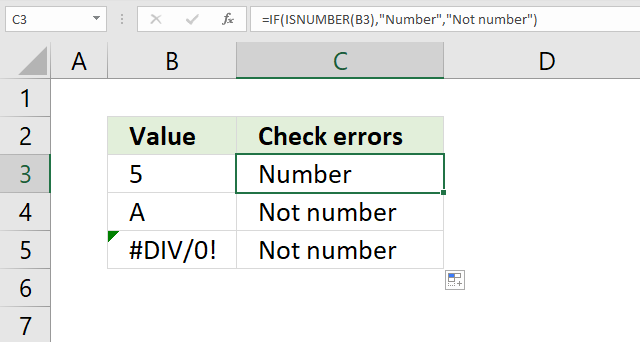The image above shows an IF function in cell C3 that checks if the adjacent cell on the same row in column B is a text value. The formula returns "Text" if true and "Not text" if false.

Formula in cell C3:

=IF(ISNUMBER(B3),"Text","Not text")

Cell reference B3 is a relative cell reference meaning it changes accordingly when you copy cell C3 and pastes it to cells below.

Explaining formula in cell C3

Step 1 - Logical expression

The ISNUMBER function returns TRUE if the argument is a number and FALSE if not.

ISNUMBER(B3)

becomes

ISNUMBER(5)

and returns boolean TRUE.

Step 2 - Evaluate IF function

IF(ISTEXT(B3), "Text", "Not text")

becomes

IF(False, "Text", "Not text")

and returns "Not text" in cell C3.

9. IF function - if false do nothing?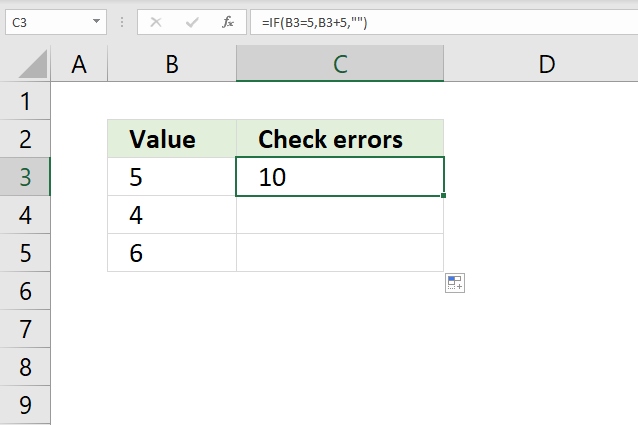The formula in cell C3 checks if the value in cell B3 is equal to 5, if so add 5 to the number in cell B3. If cell B3 is not equal to 5 do nothing.

Formula in cell C3:

=IF(B3=5,B3+5,"")

Cell reference B3 is a relative cell reference meaning it changes accordingly when you copy cell C3 and pastes it to cells below.

Explaining formula in cell C3

Step 1 - Logical expression

The equal sign lets you compare two or more values, the expression returns TRUE or FALSE.

B3=5

becomes

5=5

and returns TRUE.

Step 2 - Evaluate IF function

The second argument is returned if the logical expression returns TRUE and the third argument is returned if FALSE. The third argument is "" which is nothing. The cell is empty. The IF function returns 0 (zero) if you leave the third argument empty.

IF(B3=5,B3+5,"")

becomes

IF(TRUE,B3+5,"")

becomes

IF(TRUE,5+5,"")

and returns 10 in cell C3.

10. IF function - greater than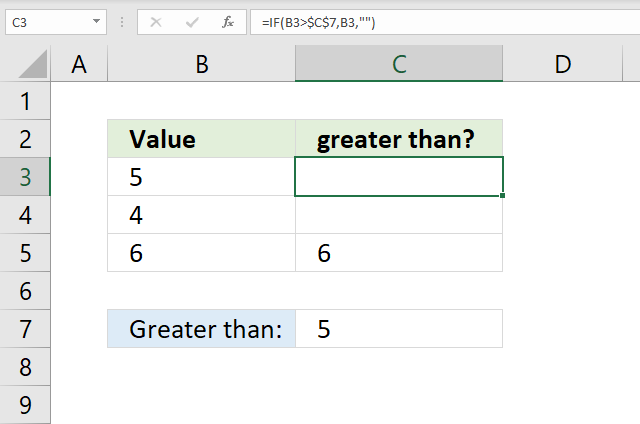The formula in cell C3 checks if the value in cell B3 is larger than cell C7, if so return the number in cell B3. If cell B3 is not larger than cell C7 then do nothing.

Formula in cell C3:

=IF(B3>\$C\$7, B3, "")

Cell reference B3 is a relative cell reference meaning it changes accordingly when you copy cell C3 and pastes it to cells below.

Explaining formula in cell C3

Step 1 - Logical expression

The larger than character lets you check if cell B3 is larger than cell C7, it returns TRUE if so. FALSE if not.

B3>\$C\$7

becomes

5>5

and returns FALSE.

Step 2 - Evaluate IF function

IF(B3>\$C\$7, B3, "")

becomes

IF(FALSE, B3, "")

becomes

IF(FALSE, 5, "")

and returns "" (nothing).

11. IF function - smaller than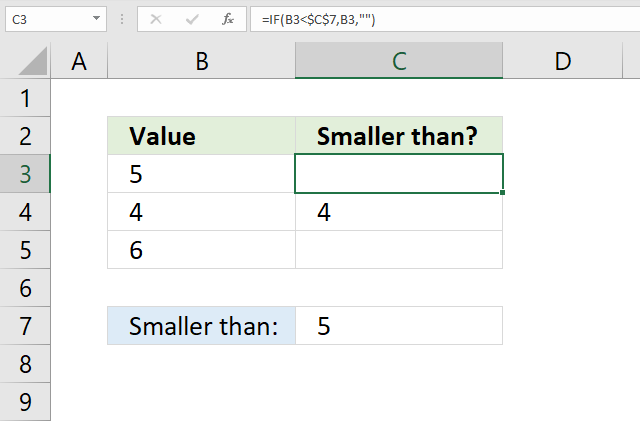The formula in cell C3 checks if the value in cell B3 is smaller than cell C7, if so return the number in cell B3. If cell B3 is not smaller than cell C7 then do nothing.

Formula in cell C3:

=IF(B3<\$C\$7, B3, "")

Cell reference B3 is a relative cell reference meaning it changes accordingly when you copy cell C3 and pastes it to cells below.

Explaining formula in cell C3

Step 1 - Logical expression

The smaller than character lets you check if cell B3 is smaller than cell C7, it returns TRUE if so. FALSE if not.

B3<\$C\$7

becomes

5<5

and returns FALSE.

Step 2 - Evaluate IF function

IF(B3<\$C\$7, B3, "")

becomes

IF(FALSE, B3, "")

becomes

IF(FALSE, 5, "")

and returns "" (nothing).

12. IF function - equals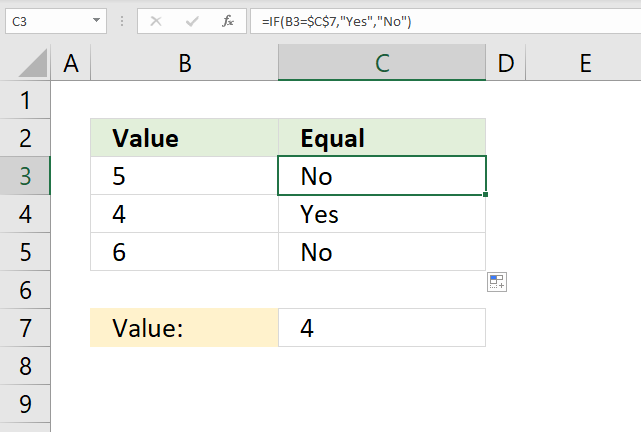The formula in cell C3 checks if the value in cell B3 is equal to value in cell C7, if so return "Yes". If cell B3 is not equal to cell C7 then return "No".

Formula in cell C3:

=IF(B3=\$C\$7, "Yes", "No")

Cell reference B3 is a relative cell reference meaning it changes accordingly when you copy cell C3 and pastes it to cells below.

Explaining formula in cell C3

Step 1 - Logical expression

The equal character lets you check if cell B3 is equal to the value in cell C7, it returns TRUE if so. FALSE if not.

B3=\$C\$7

becomes

5=4

and returns FALSE.

Step 2 - Evaluate IF function

IF(B3=\$C\$7, "Yes", "No")

becomes

IF(FALSE, "Yes", "No")

becomes

IF(FALSE, "Yes", "No")

and returns "No".

13. IF function - cell equals any of multiple values (OR logic)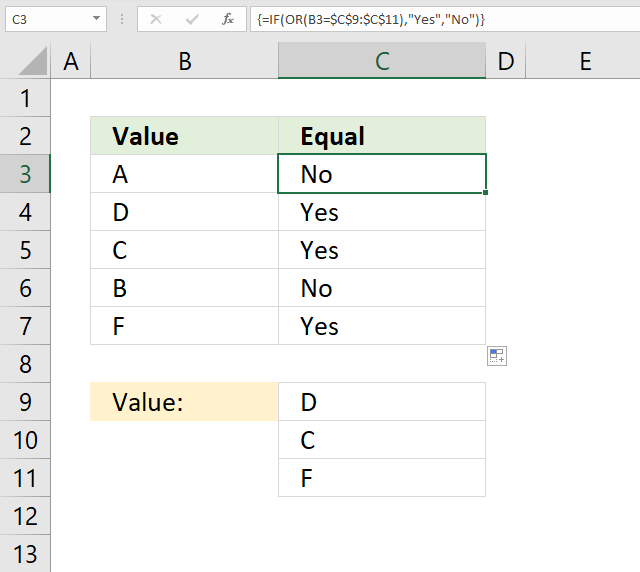The formula in cell C3 checks if the value in cell B3 is equal to any of the values in cell range C9:C11. If one value is equal then the formula returns "Yes" if not "No".

Formula in cell C3:

=IF(OR(B3=\$C\$7:\$C\$11), "Yes", "No")

Cell reference B3 is a relative cell reference meaning it changes accordingly when you copy cell C3 and pastes it to cells below.

Explaining formula in cell C3

Step 1 - Logical expression

You can use logical operators to compare one value to multiple values, the result is an array with multiple boolean values TRUE or FALSE.

B3=\$C\$7:\$C\$11

becomes

"A"={"D";"C";"F"}

and returns

{FALSE; FALSE; FALSE}. "A" is not equal to "D", "C" or "F".

Step 2 - Evaluate OR function

The OR function returns TRUE if at least one boolean value in the array is TRUE. The OR function returns FALSE if all values in the array are FALSE.

OR(B3=\$C\$7:\$C\$11)

becomes

OR({FALSE; FALSE; FALSE})

and returns FALSE.

Step 3 - Evaluate IF function

IF(OR(B3=\$C\$7:\$C\$11), "Yes", "No")

becomes

IF(FALSE, "Yes", "No")

and returns "No".

14. IF function - does not equal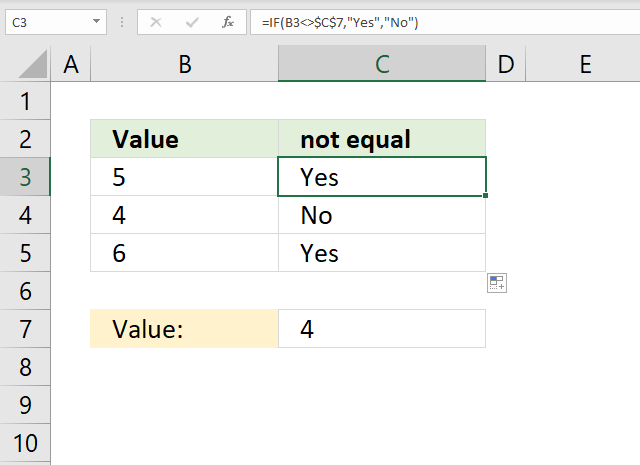The formula in cell C3 checks if the value in cell B3 is not equal to the value in cell C7, if so return "Yes". If cell B3 is not equal to cell C7 then return "No".

Formula in cell C3:

=IF(B3<>\$C\$7, "Yes", "No")

Cell reference B3 is a relative cell reference meaning it changes accordingly when you copy cell C3 and pastes it to cells below.

Explaining formula in cell C3

Step 1 - Logical expression

The less and greater than characters combined lets you check if cell B3 is not equal to the value in cell C7, it returns TRUE if so. FALSE if not.

B3<>\$C\$7

becomes

5<>4

and returns FALSE.

Step 2 - Evaluate IF function

IF(B3<>\$C\$7, "Yes", "No")

becomes

IF(TRUE, "Yes", "No")

and returns "Yes".

15. IF function - begins with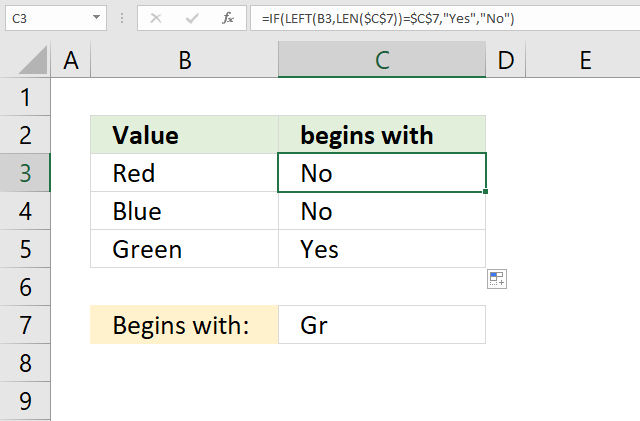The formula in cell C3 checks if the value in cell B3 begins with the value in cell C7, if so return "Yes". If cell B3 does not end with value in cell C7 then return "No".

Note that the equal sign is not evaluating text strings with regard to upper and lower letters, in other words, the equal sign is not case sensitive.

Formula in cell C3:

=IF(LEFT(B3, LEN(\$C\$7))=\$C\$7, "Yes", "No")

Cell reference B3 is a relative cell reference meaning it changes accordingly when you copy cell C3 and pastes it to cells below.

Explaining formula in cell C3

Step 1 - Count characters

The LEN function counts the number of characters in a string.

LEN(\$C\$7)

becomes

LEN("Gr")

and returns 2.

Step 2 - Return k-th characters from left

The LEFT function returns a given number of characters from left.

LEFT(B3, LEN(\$C\$7))

becomes

LEFT("Red", 2)

and returns "Re".

Step 3 - Logical test

LEFT(B3, LEN(\$C\$7))=\$C\$7

becomes

"Re"="Gr"

and returns FALSE.

Step 4 - Evaluate IF function

IF(LEFT(B3, LEN(\$C\$7))=\$C\$7, "Yes", "No")

becomes

IF(FALSE, "Yes", "No")

and returns "No" in cell C3.

16. IF function - ends with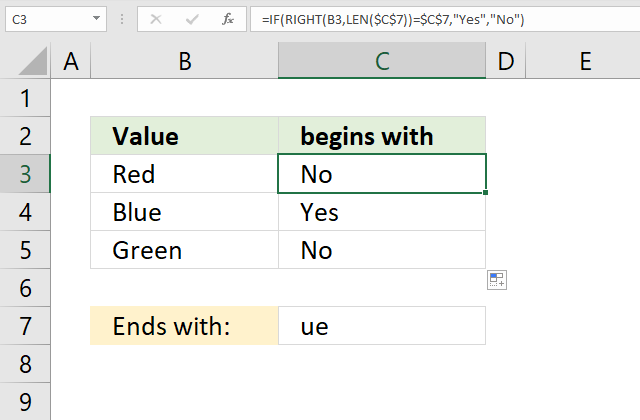The formula in cell C3 checks if the value in cell B3 ends with the value in cell C7, if so return "Yes". If cell B3 does not end with value in cell C7 then return "No".

Formula in cell C3:

=IF(RIGHT(B3, LEN(\$C\$7))=\$C\$7, "Yes", "No")

Cell reference B3 is a relative cell reference meaning it changes accordingly when you copy cell C3 and pastes it to cells below.

Explaining formula in cell C3

Step 1 - Count characters

The LEN function counts the number of characters in a string.

LEN(\$C\$7)

becomes

LEN("ue")

and returns 2.

Step 2 - Return k-th characters from left

The RIGHT function returns a given number of characters from the right.

RIGHT(B3, LEN(\$C\$7))

becomes

RIGHT("Red", 2)

and returns "ed".

Step 3 - Logical test

RIGHT(B3, LEN(\$C\$7))=\$C\$7

becomes

"ed"="ue"

and returns FALSE.

Step 4 - Evaluate IF function

IF(RIGHT(B3, LEN(\$C\$7))=\$C\$7, "Yes", "No")

becomes

IF(FALSE, "Yes", "No")

and returns "No" in cell C3.

17. IF function - cell contains a given string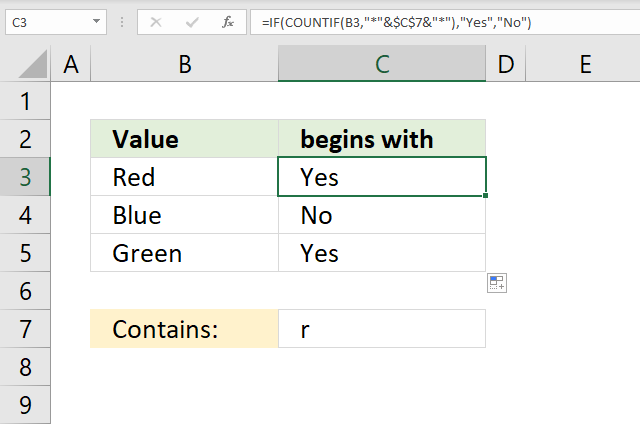The formula in cell C3 checks if the value in cell B3 contains the string in cell C7, if so return "Yes". If cell B3 does not contain the string in cell C7 then return "No".

Formula in cell C3:

=IF(COUNTIF(B3,"*"&\$C\$7&"*"),"Yes","No")

Cell reference B3 is a relative cell reference meaning it changes accordingly when you copy cell C3 and pastes it to cells below.

Explaining formula in cell C3

Step 1 - Check if value contains string

The COUNTIF function allows you to count cells matching a specific value, however, it also allows you to count cells containing a specific string.

You need to append the asterisk character before and after the string.

COUNTIF(B3, "*"&\$C\$7&"*")

becomes

COUNTIF("Red", "*r*")

and returns 1. This means that the string is found in cell B3. 1 is equal to TRUE and 0 (zero) is equal to FALSE.

Step 2 - Evaluate IF function

IF(COUNTIF(B3, "*"&\$C\$7&"*"), "Yes", "No")

becomes

IF(1, "Yes", "No")

and returns "Yes" in cell C3.

18. IF function - contains any text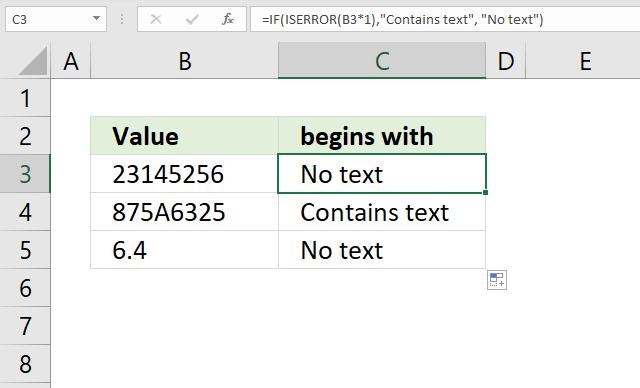The formula in cell C3 checks if the value in cell B3 is a number by multiplying with 1, if so return "Yes". If cell B3 does not contain the string in cell C7 then return "No".

Formula in cell C3:

=IF(ISERROR(B3*1),"Contains text", "No text")

Cell reference B3 is a relative cell reference meaning it changes accordingly when you copy cell C3 and pastes it to cells below.

Explaining formula in cell C3

Step 1 - Check if value contains a text string

We get an error if we try to multiply a text string with a number, we can use that technique to identify numerical values.

B3*1

becomes

23145256*1

and returns 23145256.

Step 2 - Check error

The ISERROR function returns TRUE if argument is an error value and FALSE if not.

ISERROR(B3*1)

becomes

ISERROR(23145256)

and returns FALSE.

Step 3 - Evaluate IF function

IF(ISERROR(B3*1),"Contains text", "No text")

becomes

IF(FALSE,"Contains text", "No text")

and returns "No text".

19. IF function - Exact match case sensitive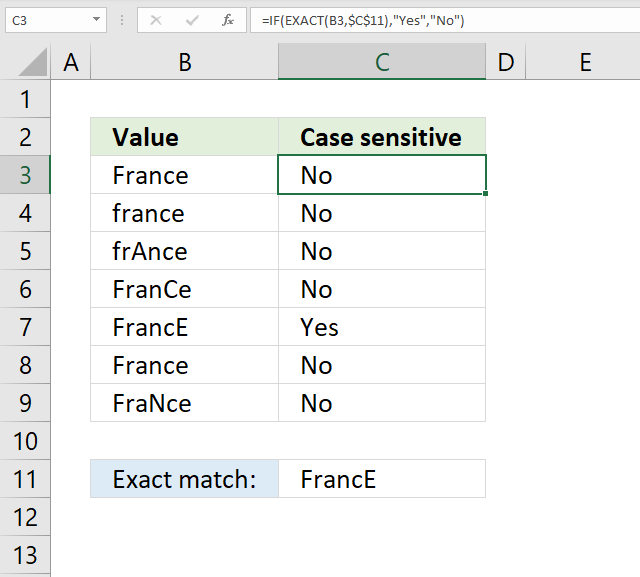The formula in cell C3 checks if the value in cell B3 is equal to the value in cell C11, upper and lower case letters are also evaluated. If a case sensitive match is found then return "Yes" otherwise return "No".

Formula in cell C3:

=IF(EXACT(B3,\$C\$11), "Yes", "No")

Cell reference B3 is a relative cell reference meaning it changes accordingly when you copy cell C3 and pastes it to cells below.

Explaining formula in cell C3

Step 1 - Check if value equals text string

The EXACT function checks if two values are precisely the same, it returns TRUE or FALSE. The EXACT function also considers upper case and lower case letters when evaluating arguments.

EXACT(B3,\$C\$11)

becomes

EXACT("France","FrancE")

and returns FALSE.

Step 2 - Evaluate IF function

IF(EXACT(B3,\$C\$11), "Yes", "No")

becomes

IF(FALSE, "Yes", "No")

and returns "No" in cell C3.

20. IF function - AND logic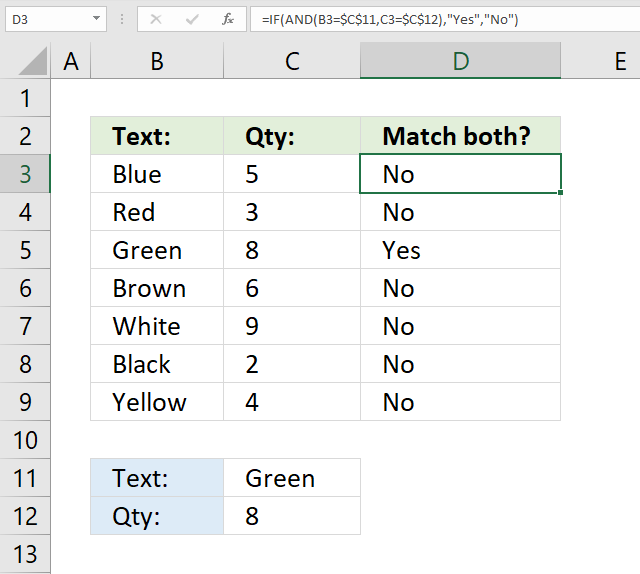The image above shows a formula in cell D3 that checks if the value in cell B3 is equal to the value in cell C11 and C3 is equal to C12. Both conditions must be met to return "Yes" otherwise return "No".

Formula in cell D3:

=IF(AND(B3=\$C\$11,C3=\$C\$12),"Yes","No")

Cell reference B3 and C3 are relative cell references meaning they change accordingly when you copy cell D3 and pastes it to cells below.

Explaining formula in cell C3

Step 1 - Evaluate first logical expression

B3=\$C\$11

becomes

"Blue"="Green"

and returns FALSE.

Step 2 - Evaluate second logical expression

C3=\$C\$12

becomes

5=8

and returns FALSE.

Step 3 - Evaluate AND function

The AND function returns TRUE if all arguments return TRUE.

AND(B3=\$C\$11,C3=\$C\$12)

becomes

AND(FALSE, FALSE)

and returns FALSE.

Step 4 - Evaluate IF function

IF(AND(B3=\$C\$11,C3=\$C\$12),"Yes","No")

becomes

IF(FALSE,"Yes","No")

and returns "No" in cell D3.

21. How to use the IF function between two worksheets?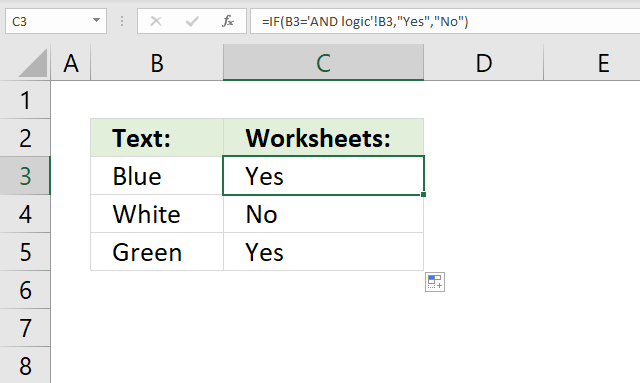The image above shows a formula in cell C3 that checks if the value in cell B3 is equal to the value in cell B3 in worksheet 'AND logic'. Note that single quotation marks are used if the worksheet name contains a space character.

Formula in cell D3:

=IF(B3='AND logic'!B3,"Yes","No")

Cell reference B3 is a relative cell references meaning it changes accordingly when you copy cell C3 and pastes it to cells below.

Note, there is an exclamation mark between the worksheet name and the cell reference.

Explaining formula in cell C3

Step 1 - Evaluate logical expression

'AND logic'!B3 is a cell reference pointing to cell B3 in worksheet 'AND logic'.

B3='AND logic'!B3

becomes

"Blue"="Blue"

and returns TRUE.

Step 2 - Evaluate IF function

IF(B3='AND logic'!B3, "Yes", "No")

becomes

IF(TRUE, "Yes", "No")

and returns "Yes" in cell D3.

22. IF function - copy cell value?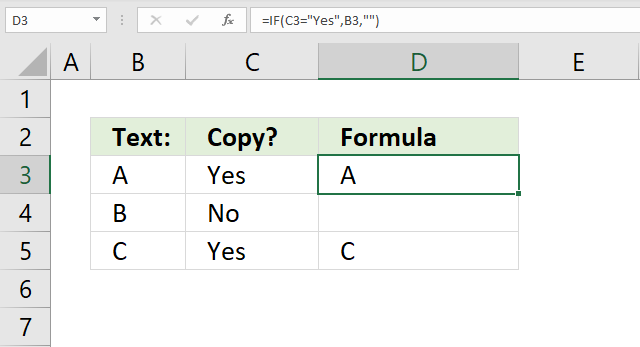The image above shows a formula in cell D3 that copies cell B3 if cell C3 is equal to "Yes". Nothing is returned if not equal to "Yes".

Formula in cell D3:

=IF(C3="Yes",B3,"")

Cell reference B3 and C3 are relative cell references meaning they change accordingly when you copy cell D3 and pastes it to cells below.

Explaining formula in cell C3

Step 1 - Evaluate logical expression

C3="Yes"

becomes

"Yes"="Yes"

and returns TRUE.

Step 2 - Evaluate IF function

IF(C3="Yes",B3,"")

becomes

IF(TRUE, "A", "")

and returns "A" in cell D3.

23. IF function - add value?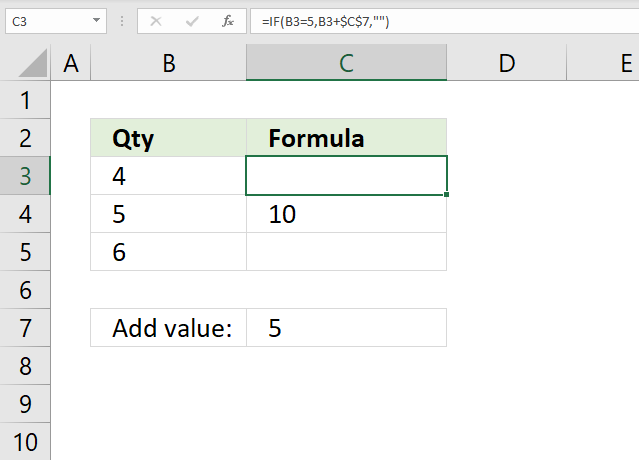The image above shows a formula in cell C3 that adds the value in cell C7 to cell B3 if cell B3 is equal to 5. Nothing "" is returned if not equal to 5.

Formula in cell D3:

=IF(B3=5,B3+\$C\$7,"")

Cell reference B3 is a relative cell references meaning it changes accordingly when you copy cell C3 and pastes it to cells below.

Explaining formula in cell C3

Step 1 - Evaluate logical expression

B3=5

becomes

4=5

and returns FALSE.

Step 2 - Evaluate IF function

IF(B3=5,B3+\$C\$7,"")

becomes

IF(FALSE,B3+\$C\$7,"")

becomes

IF(FALSE,4+5,"")

becomes

IF(FALSE,9,"")

and returns "" (nothing).

Check out the running total here: How to create a running total (SUM function)?

24. IF function - keep value?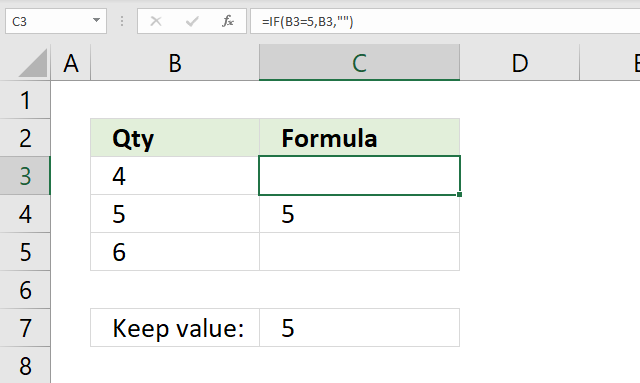The image above shows a formula in cell C3 that keeps the value in cell B3 if the value is equal to a condition.

Formula in cell D3:

=IF(B3=5,B3,"")

Cell reference B3 is a relative cell references meaning it changes accordingly when you copy cell C3 and pastes it to cells below.

Explaining formula in cell C3

Step 1 - Evaluate logical expression

B3=5

becomes

4=5

and returns FALSE.

Step 2 - Evaluate IF function

IF(B3=5,B3,"")

becomes

IF(FALSE,4,"")

and returns "" (nothing) in cell C3.

25. IF function - keep blank?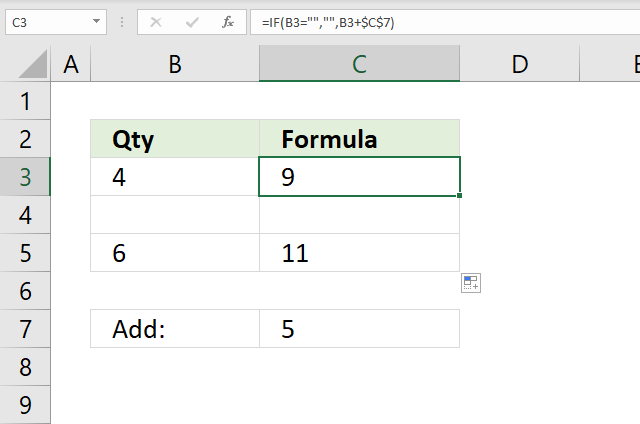The image above shows a formula in cell C3 that keeps the blank, if no blank the value in cell C7 is added to the value in column B.

Formula in cell D3:

=IF(B3="","", B3+\$C\$7)

Cell reference B3 is a relative cell references meaning it changes accordingly when you copy cell C3 and pastes it to cells below.

Explaining formula in cell C3

Step 1 - Evaluate logical expression

B3=""

becomes

4=""

and returns FALSE.

Step 2 - Evaluate IF function

IF(B3="","", B3+\$C\$7)

becomes

IF(FALSE,"", 4+5)

becomes

IF(FALSE,9,"")

and returns 9 in cell C3.

26. If function - based on cell color?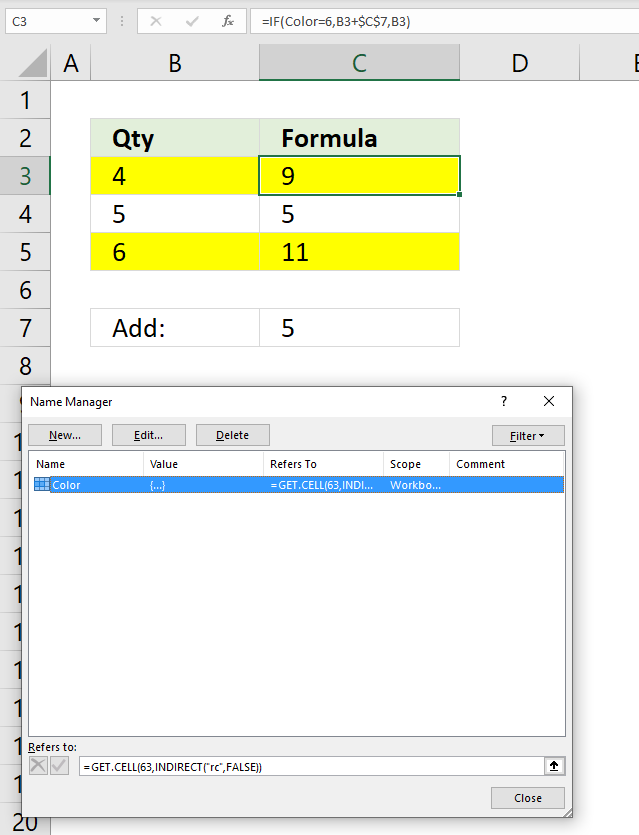The image above shows a formula in cell C3 that checks the cell background color and if color is equal to yellow add value in cell C7 to cell B3 and return the sum to cell C3..

You must first create a named range in order to use the GET.CELL function. Here is how:

1. Go to tab "Formulas" on the ribbon.
2. Press with left mouse button on "Name Manager" button.
3. A dialog box appears. Press with left mouse button on "New..." button.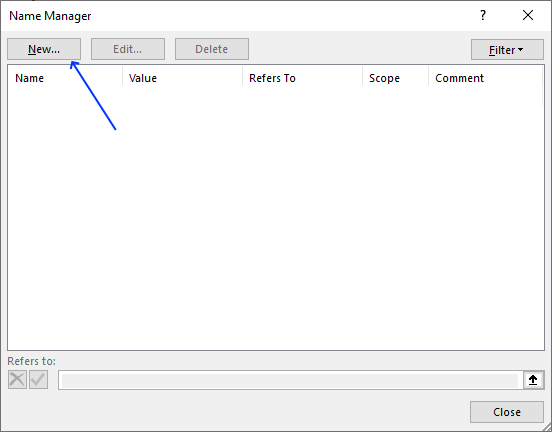4. Another dialog box appears.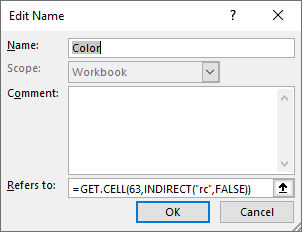5. Enter a name, I chose Color.
6. Type the following formula in "Refers to:"
=GET.CELL(63,INDIRECT("rc",FALSE))
7. Press with left mouse button on the OK button.
8. Press with left mouse button on the "Close" button.

Now back to the worksheet, formula in cell C3:

=IF(Color=6,B3+\$C\$7,B3)

Cell reference B3 is a relative cell references meaning it changes accordingly when you copy cell C3 and pastes it to cells below.

Note, the GET.CELL function has some serious flaws:

1. Obsolete and unsupported.
2. Microsoft may remove the GET.CELL function whenever they feel like it.
3. It does not update when a cell background-color is changed, you need to press F9 to recalculate the formulas.
4. It does not support all the millions of colors available in Excel, only around 60 colors.

I recommend that you use Conditional Formatting to color cells based on a condition and use the same condition in the IF function.

Here is an example: How to sum by color?

Explaining formula in cell C3

Step 1 - Identify cell background-color

Color=6

becomes

6=6

and returns TRUE. A yellow background-color returns 6.

Step 2 - Evaluate IF function

IF(Color=6,B3+\$C\$7,B3)

becomes

IF(TRUE, 4+5,4)

and returns 9. 4+5 = 9.

27. How to work with multiple values in the IF function? (arrays)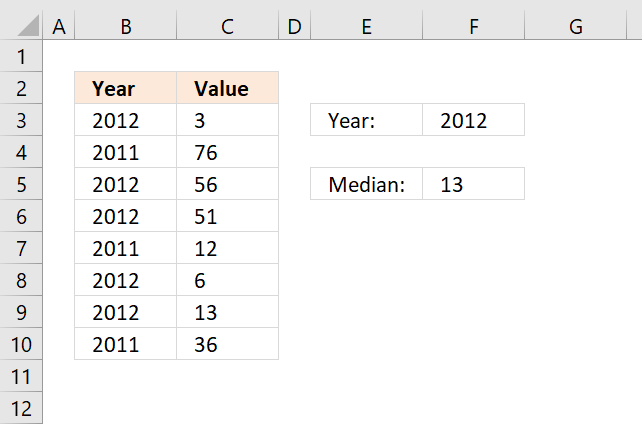The image above demonstrates a formula in cell F5 that uses the specified value in cell F3 to filter values from column C based on column B.

If the year in column B matches the value in cell F3 the corresponding value on the same row is used. The formula is actually performing this calculation to all values in cell range B3:B10.

Array formula in cell F5:

=MEDIAN(IF(B3:B10=F3,C3:C10,""))

This is an array formula, to enter an array formula press and hold CTRL + SHIFT simultaneously after you have entered the formula in a cell. Press Enter once and then release all keys.

The formula changes and now has curly brackets surrounding the formula, like this: {=MEDIAN(IF(B3:B10=F3,C3:C10,""))}

Do not enter these characters yourself, they appear automatically. Note that Office 365 subscribers do not need to enter this as an array formula, simply enter it as a regular formula. This is a new feature in Excel called Dynamic arrays.

Explaining formula in cell F5

Step 1 -  Logical expression

B3:B10=F3

becomes

{2012; 2011; 2012; 2012; 2011; 2012; 2012; 2011}=2012

and returns

{TRUE; FALSE; TRUE; TRUE; FALSE; TRUE; TRUE; FALSE}

Step 2 - Evaluate IF function

The IF function compares all values in cell range B3:B10 to the value in cell F3 and returns the corresponding value from cell range C3:C10 if they match. Nothing "" is returned if they don't match.

IF(B3:B10=F3,C3:C10,"")

becomes

IF({TRUE; FALSE; TRUE; TRUE; FALSE; TRUE; TRUE; FALSE}, C3:C10, "")

becomes

IF({TRUE; FALSE; TRUE; TRUE; FALSE; TRUE; TRUE; FALSE}, {3; 76; 56; 51; 12; 6; 13; 36}, "")

and returns {3; ""; 56; 51; ""; 6; 13; ""}.

Step 3 - MEDIAN function

The MEDIAN function returns the middle value from the array.

MEDIAN(IF(B3:B10=F3,C3:C10,""))

becomes

MEDIAN({3; ""; 56; 51; ""; 6; 13; ""})

and returns 13 in cell F5.

28. IF function - between two numbers?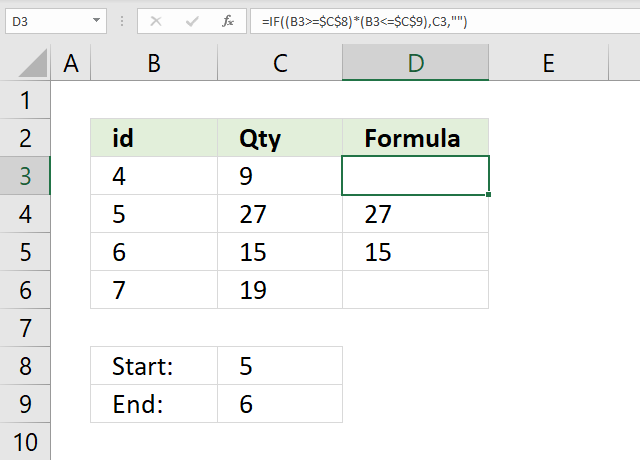The image above shows a formula in cell D3 that checks if number in cell B3 is between two numbers specified in cell C8 and C9. If not in range then return nothing "".

Formula in cell D3:

=IF((B3>=\$C\$8)*(B3<=\$C\$9), C3, "")

Cell reference B3 is a relative cell references meaning it changes accordingly when you copy cell C3 and pastes it to cells below.

Explaining formula in cell C3

Step 1 - Evaluate first logical expression

B3>=\$C\$8

becomes

4>=5

and returns FALSE.

Step 2 - Evaluate second logical expression

B3<=\$C\$9

becomes

4<=6

and returns TRUE.

Step 3 - Multiply logical expressions (AND logic)

The parentheses are needed to control the order of operation. We need to compare values first before multiplying boolean values.

(B3>=\$C\$8)*(B3<=\$C\$9)

becomes

FALSE*TRUE

and returns 0 (zero) which is equivalent to FALSE.

Step 4 - Evaluate IF function

IF((B3>=\$C\$8)*(B3<=\$C\$9), C3, "")

becomes

IF(0, C3, "")

and returns "" (nothing) in cell D3.

29. IF function - based on date?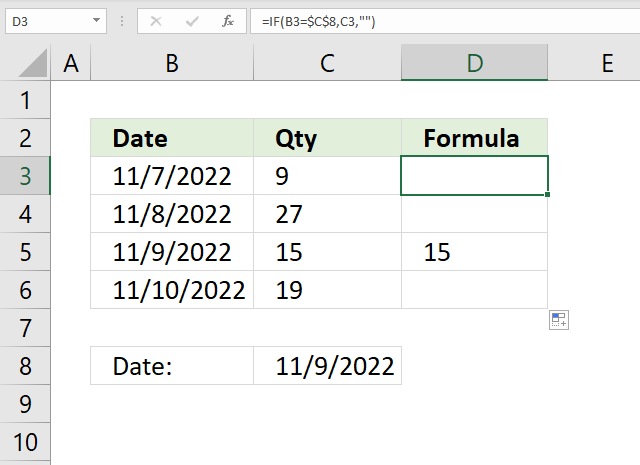The image above shows a formula in cell D3 that checks if the date in cell B3 is equal to the date in cell C8, if so return the number in cell C3.. If not then return nothing "".

Formula in cell D3:

=IF(B3=\$C\$8,C3,"")

Cell reference B3 is a relative cell references meaning it changes accordingly when you copy cell C3 and pastes it to cells below.

Note, keep in mind that dates in Excel are actually numbers formatted as dates. This makes it possible to add or subtract days, months, years, hours, and seconds to a date.

For example, number 1 is 1/1/1900 and 1/1/2000 is 36526. You can see that this is correct, type 1 in a cell. Select the cell, press CTRL + 1 to format the cell. Press with left mouse button on Date and then press OK button.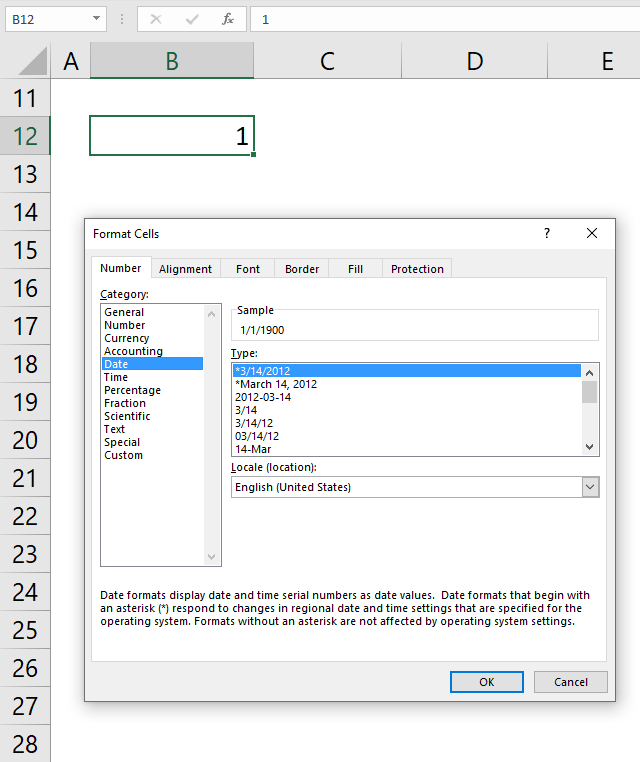Explaining formula in cell D3

Step 1 - Compare dates

B3=\$C\$8

becomes

44872=44874

and returns FALSE.

Step 2 - Evaluate IF function

IF(B3=\$C\$8,C3,"")

becomes

IF(FALSE,44872,"")

and returns "" (nothing) in cell D3.